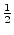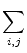# Non smooth geodesic flows and classical mechanics

Marsden, J. E.

Canad. Math. Bull., 12, (1969), 209-212

### Abstract:

As is well known, there is an intimate connection between geodesic flows and Hamiltonian systems. In face, if g is a Riemannian, or pseudo-Riemannian metric on a manifold M (we think of M as q -space or the configuration space), we may define a smooth function Tg on the cotangent bundle T*M (q -p -space, or phase space). This function is the kinetic energy of q , and locally is given by

Tg(q, p) =gij(qPi Pj

Where q = (q1,..., qn) and p = (p1,..., pn) and g has components gij .

Using Tg as a Hamiltonian function, the associated flow (that is, the global solution of Hamiltonian's equations) is exactly the geodesic flow; geodesics are obtained by projection to M .

Conversely, Hamiltonian motion in a potential V and metric g , that is, H = Tg + V , may be thought of as geodesic motion using the metric (e - V)g if e > V(q) . This new metric is called the Jacobi metric.

Traditionally, the theory of classical mechanics and Riemannian geometry always assumes g and V are smooth functions. However, the most elementary examples in fact are not smooth. One of the main reasons for the smoothness assumption was to guarantee existence of the flow (geodesics). This objection has now been removed.

The purpose of this note is to explain in an expository fashion what changes are necessary in the above theory to cover the non-smooth case. This new situation is quite different, although some interesting observations can be made.